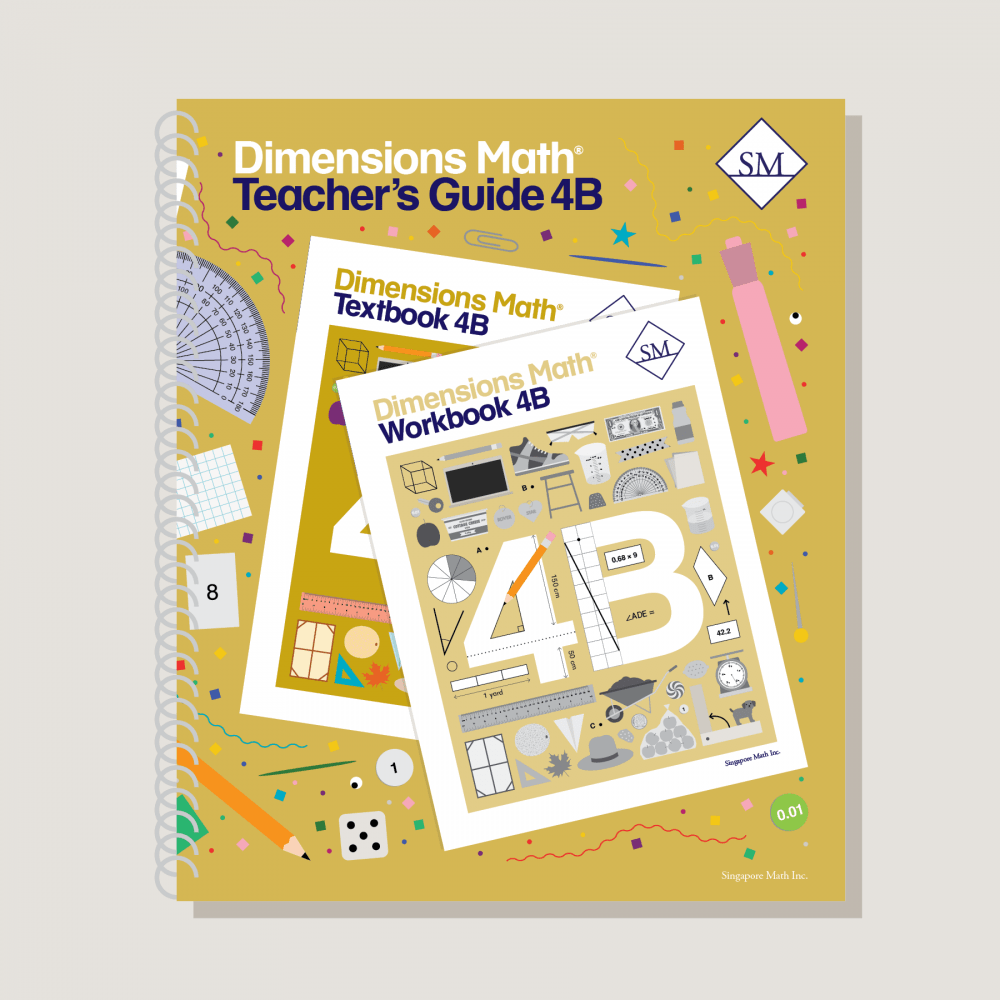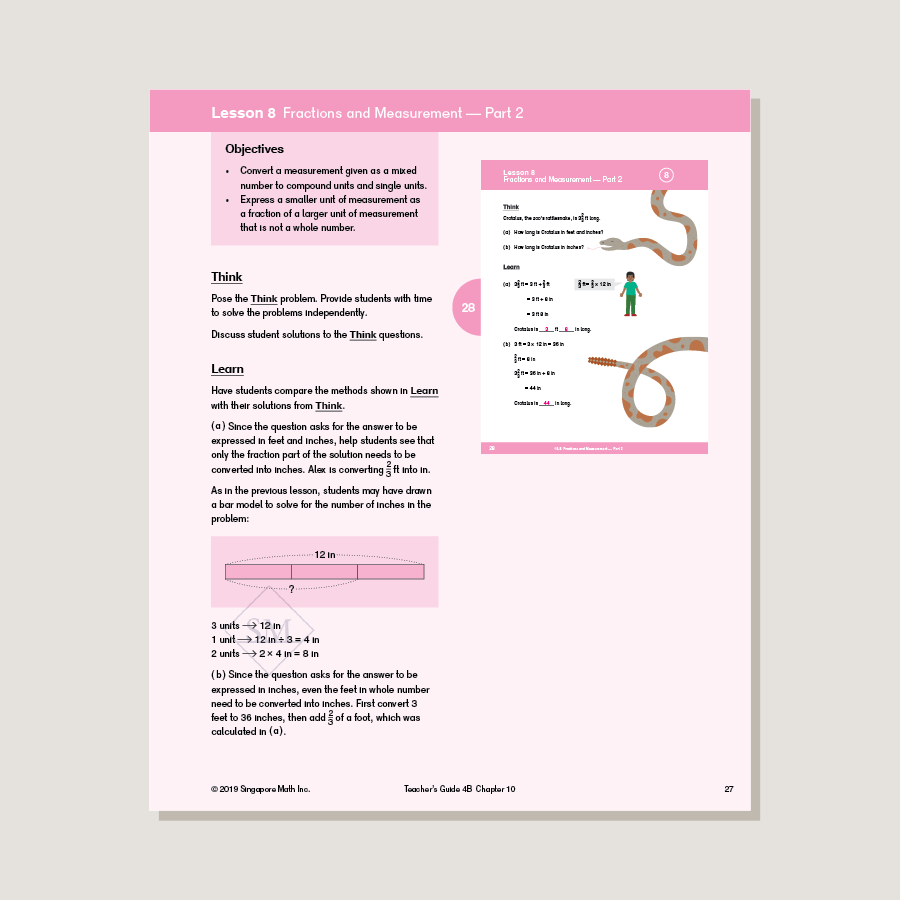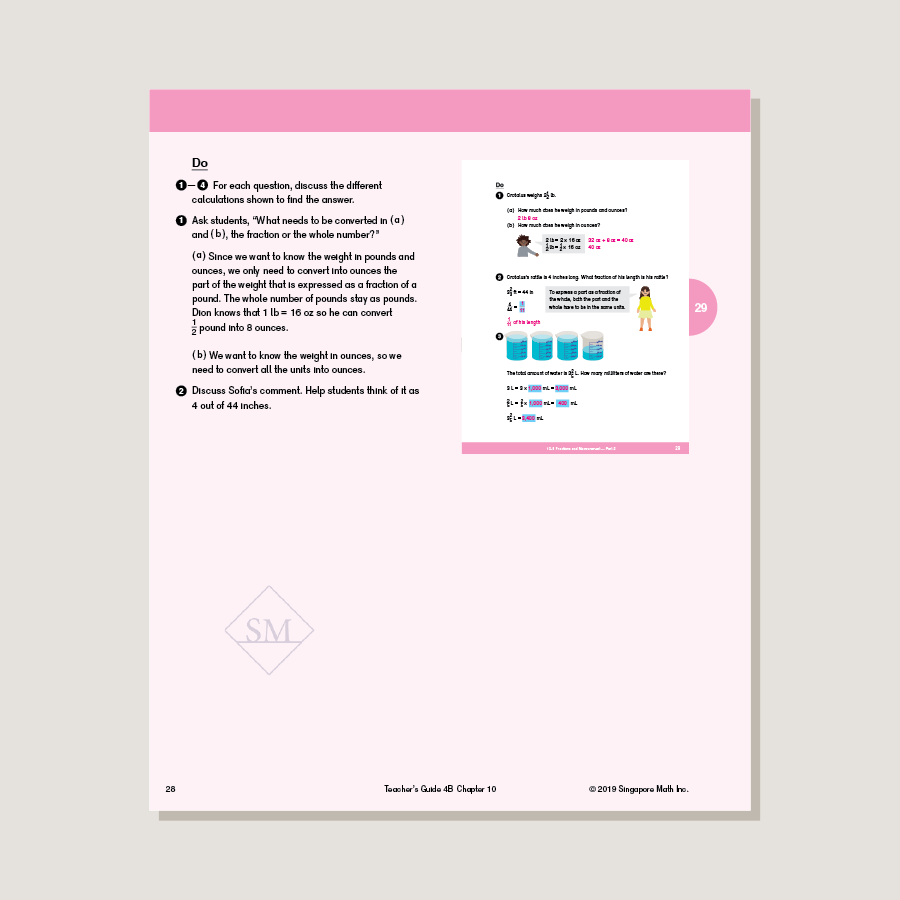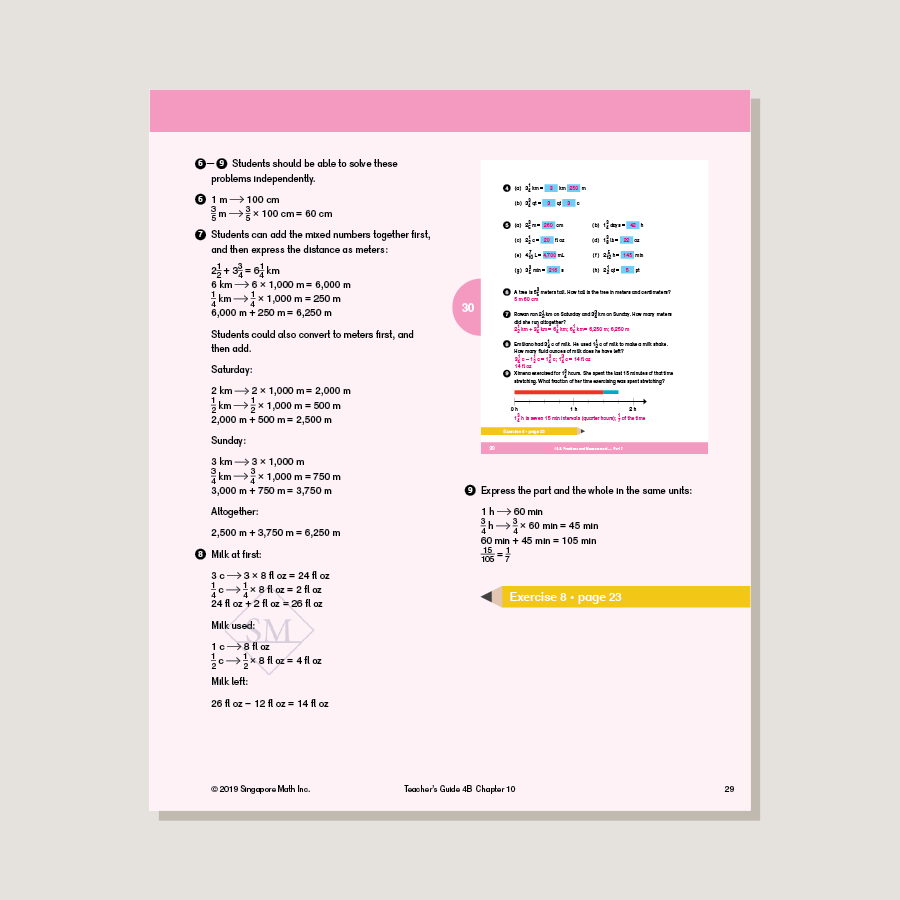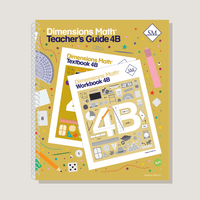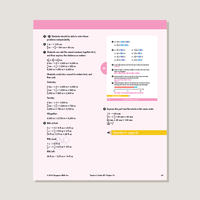# Dimensions Math Teacher's Guide 4B

\$31.50

Teacher’s guides are a comprehensive resource for achieving lesson objectives. They include background information, detailed lesson plans, helpful suggestions, and activities. They help educators understand the purpose of each lesson within the context of the curriculum. A high level of educator involvement is essential for success in this program.

Note: Hard cover. Spiral-bound.

SKU: DMTG4B

ISBN: 9781947226395

Pagecount: 278

Dimensions: 11 x 12 x 1 in

Binding: Spiral

Color: Color

Cover: Hard

Perforated: No

Sample Pages

Chapter 10: Measurement
Teaching Notes
Chapter Opener
Lesson 1: Metric Units of Measurement
Lesson 2: Customary Units of Length
Lesson 3: Customary Units of Weight
Lesson 4: Customary Units of Capacity
Lesson 5: Units of Time
Lesson 6: Practice A
Lesson 7: Fractions of Measurement — Part 1
Lesson 8: Fractions of Measurement — Part 2
Lesson 9: Practice B
Workbook Solutions

Chapter 11: Area and Perimeter
Teaching Notes
Chapter Opener
Lesson 1: Area of Rectangles Part 1
Lesson 2: Area of Rectangles Part 2
Lesson 3: Area of Composite Figures
Lesson 4: Perimeter — Part 1
Lesson 5: Perimeter — Part 2
Lesson 6: Practice
Workbook Solutions

Chapter 12: Decimals
Teaching Notes
Chapter Opener
Lesson 1: Tenths — Part 1
Lesson 2: Tenths — Part 2
Lesson 3: Hundredths — Part 1
Lesson 4: Hundredths — Part 2
Lesson 5: Expressing Decimals as Fractions in Simplest Form
Lesson 6: Expressing Fractions as Decimals
Lesson 7: Practice A
Lesson 8: Comparing and Ordering Decimals
Lesson 9: Rounding Decimals
Lesson 10: Practice B
Workbook Solutions

Chapter 13: Addition and Subtraction of Decimals
Teaching Notes
Chapter Opener
Lesson 1: Adding and Subtracting Tenths
Lesson 2: Adding Tenths with Regrouping
Lesson 3: Subtracting Tenths with Regrouping
Lesson 4: Practice A
Lesson 6: Subtracting from 1 and 0.1
Lesson 7: Subtracting Hundredths
Lesson 8: Money, Decimals, and Fractions
Lesson 9: Practice B
Review 3
Workbook Solutions

Chapter 14: Multiplication and Division of Decimals
Teaching Notes
Chapter Opener
Lesson 1: Multiplying Tenths and Hundredths
Lesson 2: Multiplying Decimals by a Whole Number — Part 1
Lesson 3: Multiplying Decimals by a Whole Number — Part 2
Lesson 4: Practice A
Lesson 5: Dividing Tenths and Hundredths
Lesson 6: Dividing Decimals by a Whole Number — Part 1
Lesson 7: Dividing Decimals by a Whole Number — Part 2
Lesson 8: Dividing Decimals by a Whole Number — Part 3
Lesson 9: Practice B
Workbook Solutions

Chapter 15: Angles
Teaching Notes
Chapter Opener
Lesson 1: The Size of Angles
Lesson 2: Measuring Angles
Lesson 3: Drawing Angles
Lesson 4: Adding and Subtracting Angles
Lesson 5: Reflex Angles
Lesson 6: Practice
Workbook Solutions

Chapter 16: Lines and Shapes
Teaching Notes
Chapter Opener
Lesson 1: Perpendicular Lines
Lesson 2: Parallel Lines
Lesson 3: Drawing Perpendicular and Parallel Lines
Lesson 5: Lines of Symmetry
Lesson 6: Symmetrical Figures and Patterns
Lesson 7: Practice
Workbook Solutions

Chapter 17: Properties of Cuboids
Teaching Notes
Chapter Opener
Lesson 1: Cuboids
Lesson 2: Nets of Cuboids
Lesson 3: Faces and Edges of Cuboids
Lesson 4: Practice
Review 4Review 5
Workbook Solutions

A & B Books: Our programs divide the school year into two semesters. “A” level books are for the first half of the school year. “B” level books are for the second half of the school year. You need both “A” and “B” material for a complete school year.

Required Components: Textbooks, Workbooks, and Guides (either Home Instructor’s Guides or Teacher’s Guides) are all necessary components. These three elements each serve a unique function and work together to build math mastery.

Printouts: Dimensions Math PK-5 Resources

Recommended Manipulatives: Dimensions Math Grades PK-5 Recommended Manipulatives